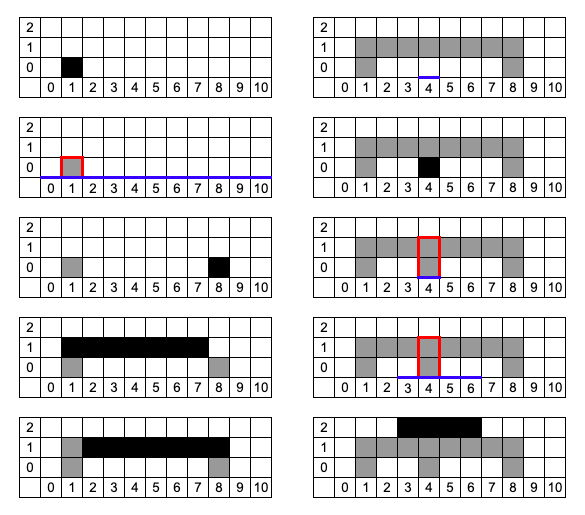시간 제한 메모리 제한 제출 정답 맞은 사람 정답 비율
12 초 1024 MB 4 1 1 100.000%

## 문제

There is an cell grid infinite in left, right, and upwards directions (all the cells with coordinates $(x, y)$ with $x \in \mathbb{Z}$, $y \ge 0$ exist). Initially all the cells are white. You have to process $q$ queries of two types:

1. $y_{i}$ $l_{i}$ $r_{i}$: paint all cells $(x, y_{i})$ for $l_{i} \le x \le r_{i}$ black. If the cell is already black, its color doesn't change.
2. $l_{i}$ $r_{i}$: consider all cells with $x$ coordinate on the segment $[l_{i}; r_{i}]$. Find the highest cell such that all cells exactly under it are black. Formally, you have to find maximal $h$ such that $\exists \: x \in [l_{i}; r_{i}] \: \forall \: y \in [0;h)$ cell $(x, y)$ is black.

To enforce processing the queries online they are encrypted using previous answers.

## 입력

The first line contains one integer $q$ ($1 \le q \le 10^{5}$) --- the number of queries to process.

The next $q$ lines will contain encrypted descriptions of queries. Let $S$ be the sum of answers to all queries of second type processed so far.

Each description has form either $1$ $(y_{i} \oplus S)$ $(l_{i} \oplus S)$ $(r_{i} \oplus S)$ or $2$ $(l_{i} \oplus S)$ $(r_{i} \oplus S)$. It is guaranteed that $0 \le y_{i} \le 2 \cdot 10^{5}$, $0 \le l_{i} \le r_{i} \le 2 \cdot 10^{5}$. Note that the guarantees are given on parameters after decryption, the numbers in input might not fit in 32-bit integers.

Don't forget to add the new answer to $S$ after each query of the second type.

## 출력

Print the answers to all queries of the second type on separate lines.

## 예제 입력 1

10
1 0 1 1
2 0 10
1 1 9 9
1 0 0 6
1 0 3 9
2 5 5
1 1 5 5
2 5 5
2 0 5
1 7 6 3


## 예제 출력 1

1
0
2
2


## 힌트

S Encrypted Query Ans
0 1 0 1 1 1 0 1 1 -
0 2 0 10 2 0 10 1
1 1 1 9 9 1 0 8 8 -
1 1 0 0 6 1 1 1 7 -
1 1 0 3 9 1 1 2 8 -
1 2 5 5 2 4 4 0
1 1 1 5 5 1 0 4 4 -
1 2 5 5 2 4 4 2
3 2 0 5 2 3 6 2
5 1 7 6 3 1 2 3 6 -